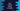# Dart program to convert a string to double## Dart program to convert string to double:

This post will show you how to convert a string to double in dart programming language. Dart provides parse and tryParse

### parse method in double class:

parse method is defined as below:

``double parse(String strValue)``

It accepts a floating point value or Infinity or NaN with a + or - at start. It should not be null. Leading and trailing whitespaces are ignored. It throws FormatException for invalid inputs.

### Example of parse method:

Let me show you a couple of examples:

``````void main() {
double double_1 = double.parse('123');
double double_2 = double.parse('2.5');
double double_3 = double.parse('.3');
double double_4 = double.parse('+3.14');
double double_5 = double.parse('+2.e3');
double double_6 = double.parse('+234E+8');
double double_7 = double.parse('-NaN');
double double_8 = double.parse('+Infinity');
double double_9 = double.parse('   12.334   ');

print("double_1 \$double_1");
print("double_2 \$double_2");
print("double_3 \$double_3");
print("double_4 \$double_4");
print("double_5 \$double_5");
print("double_6 \$double_6");
print("double_7 \$double_7");
print("double_8 \$double_8");
print("double_9 \$double_9");
}``````

These all are valid inputs. It will print the below output:

``````double_1 123.0
double_2 2.5
double_3 0.3
double_4 3.14
double_5 2000.0
double_6 23400000000.0
double_7 NaN
double_8 Infinity
double_9 12.334``````

### Exception and error:

parse method works only for valid doubles. If we pass anything less than double.minPositive or more than double.maxFinite, it will return Infinite. For any other values that can’t be parsed to double, it will throw FormatException. For null, it will throw NoSuchMethodError.

``````void main() {
try {
double double_1 = double.parse('hello');
} on FormatException {
print("FormatException for double_1");
}

try {
double double_2 = double.parse('2,34');
} on FormatException {
print("FormatException for double_2");
}

try {
double double_3 = double.parse(null);
} on NoSuchMethodError {
print("NoSuchMethodError for double_3");
}
}``````

For all of these parse statements, exception and error is thrown. If you run it, it will throw the below output:

``````FormatException for double_1
FormatException for double_2
NoSuchMethodError for double_3``````

### tryParse to parse string to double:

tryParse is another method that can be used to parse a string to double. This is defined as below:

``double? tryParse(String strValue)``

It will not throw any exception, but it will return null. Only for null, it will throw NoSuchMethodError.

For example:

``````void main() {
double double_1 = double.tryParse('123');
double double_2 = double.tryParse('2.5');
double double_3 = double.tryParse('.3');
double double_4 = double.tryParse('+3.14');
double double_5 = double.tryParse('+2.e3');
double double_6 = double.tryParse('+234E+8');
double double_7 = double.tryParse('hello');
double double_8 = double.tryParse('1.23x');

print("double_1 \$double_1");
print("double_2 \$double_2");
print("double_3 \$double_3");
print("double_4 \$double_4");
print("double_5 \$double_5");
print("double_6 \$double_6");
print("double_7 \$double_7");
print("double_8 \$double_8");
}``````

It will print:

``````double_1 123.0
double_2 2.5
double_3 0.3
double_4 3.14
double_5 2000.0
double_6 23400000000.0
double_7 null
double_8 null``````

As you can see here, it prints null for the last two.